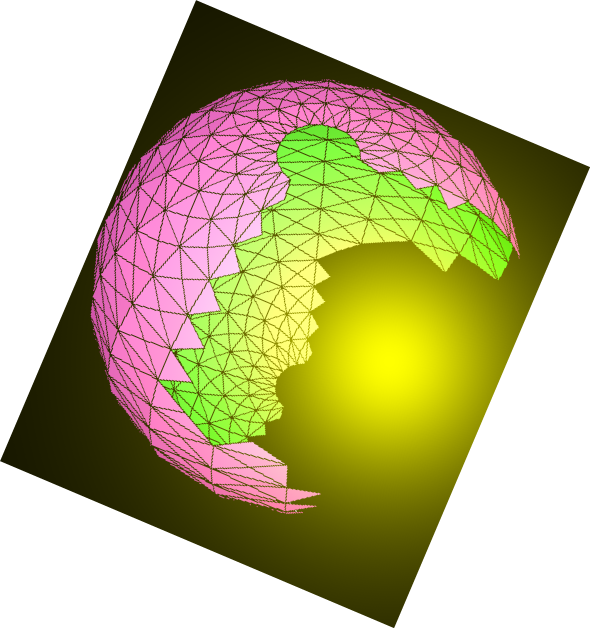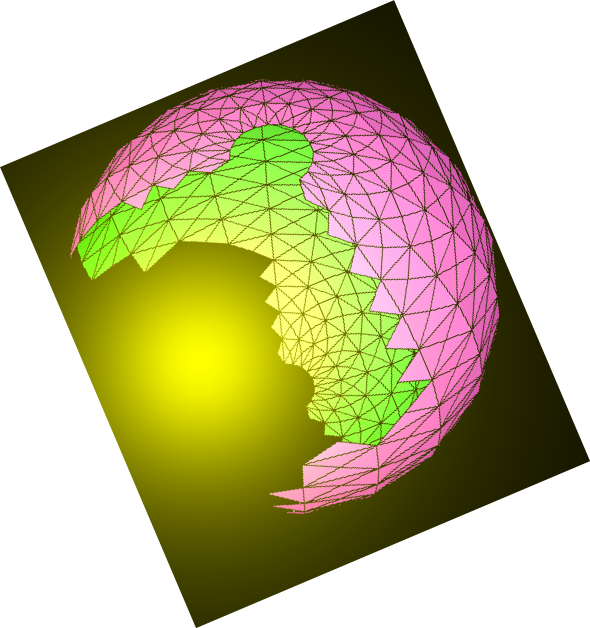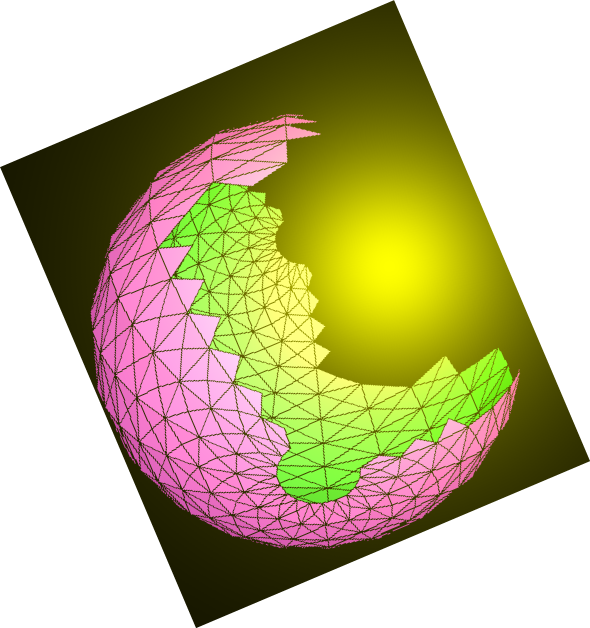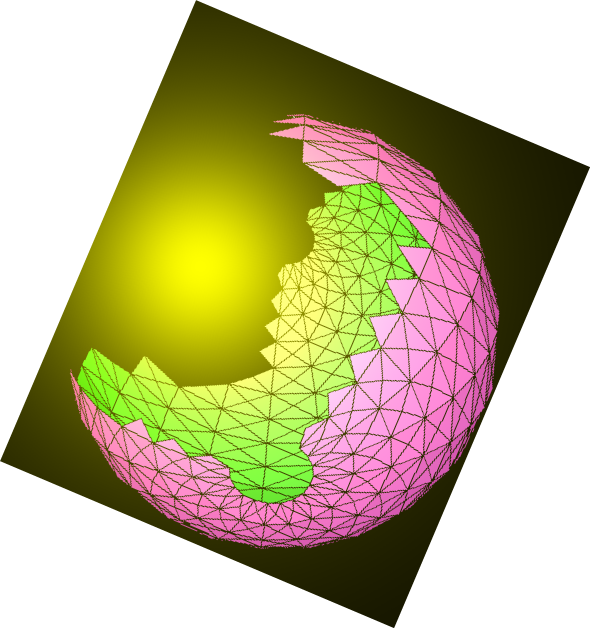# N.S.B. Cosmic Center# JavaScript & MathScript Interactive Document Generator-Demo-10

This document is generated by N.S.B. JavaScript & MathScript Interactive Document Generator version 001.

Introduction to N.S.B. JavaScript & MathScript Interactive Document Generator

MathScript Functions (cont.)

Before concluding our introduction to our JavaScript and MathScript Interactive Document Generator, and before shifting to more advanced aspects and applications of MathScript, we give a few more useful elementary functions.

The function isFree[] checks whether a MathScript function is free from some element (anywhere in its structure or substructures), that element being of a certain expression or property. For instance, consider the followings:

###### output(11)=> false

You should have noticed that we have checked whether our expression is free from mathematical expressions of addition, multiplication, and power (denoted by the function heads add, mult, and pow, respectively) and we found correct answers for that matter.

The functions unite[] and intersect[] are useful in combining the elements of two MathScript functions (having the same head expression) as if you are conctructing the union and the intersection of two sets respectively. Consider the followings:

###### output(17)=> {x, b}

Now that you have followed these demodocs up to this point, and that you have used our client-side program to verify and experiment with code, either JavaScript or MathScript, then your preliminary knowledge is adequate to start on a forthcoming set of demodocs pertaining to advanced aspects and applications.

###### NextHome
N.S.B. Cosmic Center